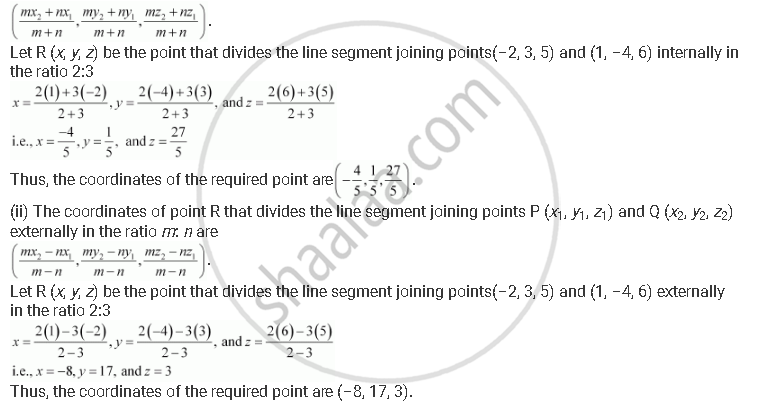CBSE (Arts) Class 11CBSE
Share

# Find the Coordinates of the Point Which Divides the Line Segment Joining the Points (–2, 3, 5) and (1, –4, 6) in the Ratio (I) 2:3 Internally, (Ii) 2:3 Externally. - CBSE (Arts) Class 11 - Mathematics

ConceptThree - Dimensional Geometry Section Formula

#### Question

Find the coordinates of the point which divides the line segment joining the points (–2, 3, 5) and (1, –4, 6) in the ratio (i) 2:3 internally, (ii) 2:3 externally.

#### Solution

(i) The coordinates of point R that divides the line segment joining points P (x1y1z1) and Q (x2y2z2) internally in the ratio mareIs there an error in this question or solution?

#### APPEARS IN

NCERT Solution for Mathematics Textbook for Class 11 (2018 to Current)
Chapter 12: Introduction to Three Dimensional Geometry
Q: 1 | Page no. 277

#### Video TutorialsVIEW ALL 

Solution Find the Coordinates of the Point Which Divides the Line Segment Joining the Points (–2, 3, 5) and (1, –4, 6) in the Ratio (I) 2:3 Internally, (Ii) 2:3 Externally. Concept: Three - Dimensional Geometry - Section Formula.
S